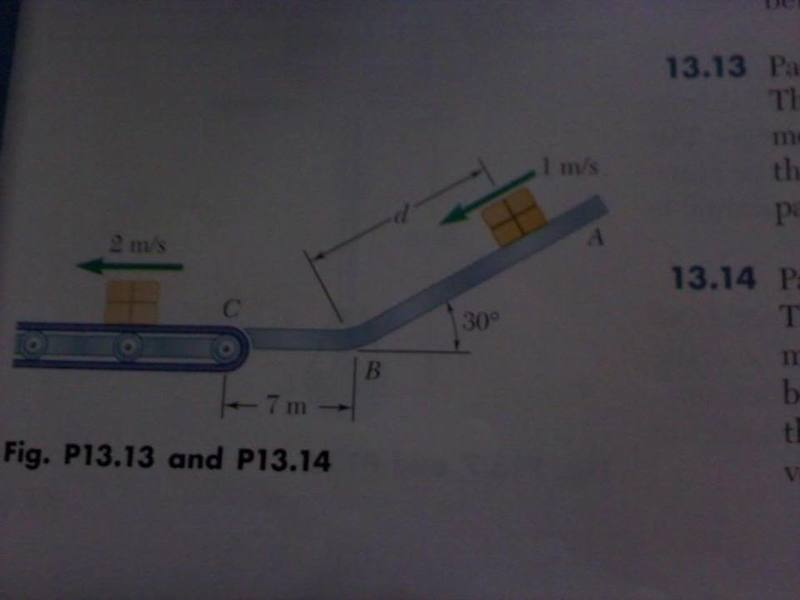# Dynamics - packages on an incline

Packages are thrown down an incline at A with a velocity of 1 m/s.
The packages slide along the surface ABS to a conveyor belt which moves with a velocity of 2 m/s.

Knowing that d = 7.5 m and µ = 0.25 between the packages and all surfaces, determine...

a) speed of the package at C
b) distance a package will slide on the conveyor belt before it comes to rest relative to the belt

Attempted solution:

FBD -- N = Wsinθ for part 1

Kinetic energy = T
Work = U

T2 = T1 + U12
T2 = 1/2 mv^2 + [Fweight - Ffriction]*distance
T2 = m*[1/2+ Wsinθ-µWcosθ]*(7.5 m)

T2 = 21.36*m (Energy at point B)

Then....

N = W = mg

T3 = T2 + U23 = T2 -(Ffriction*distance)
T3 = 21.36*m - .25*mg*7
T3 = 4.19*m

4.19*m = 1/2 mv^2
v = sqrt(4.19*2)

v=2.89 m/s

this is the correct velocity at the point, but when i try to find the distance it will slide, i cant.

TF= Tfinal = 0

Tf = T3 + U3->F = 0
T3 = -U3F
4.19*m = µ*mg*distance

distance = 4.19/(µ*g)

this gives me a distance of 1.7 m, but the answer is 0.893 m.Last edited:

tiny-tim
Homework Helper
hi pleasehelpme6!(have a mu: µ and a theta: θ and try using the X2 icon just above the Reply box)
TF= Tfinal = 0

noooo …hi pleasehelpme6!(have a mu: µ and a theta: θ and try using the X2 icon just above the Reply box)

noooo …with respect to the belt, wouldnt the final energy be zero?
or is there some momentum change i missed? (we havent covered momentum yet so i could easily have missed it)

tiny-tim
Homework Helper
with respect to the belt, wouldnt the final energy be zero?

yes, and you can do it wrt the belt …

but then the initial v won't be 2.89, will it?does that mean the package doesnt change velocity at all from touching the belt?

that would mean the velocity is .89 m/s, making T3/belt=.5*m(.89)^2

so...

Tstop = 0 = T3/belt + Wfriction
T3/belt = -Wfriction

((.5)*.89^2)/(µg) = distance = 0.16

which is incorrect.

tiny-tim
Homework Helper
i think the question is asking for the distance relative to the ground

it asks for "the distance a package will slide on the conveyor belt before it comes to rest relative to the belt"

tiny-tim
Homework Helper
it asks for "the distance a package will slide on the conveyor belt before it comes to rest relative to the belt"

maybe, maybe not …

it doesn't say "the distance a package will slide relative to the belt" …

and i think my interpretation gives the correct answerthe distance the package will slide ON THE BELT, before it comes to rest relative to the belt.
this leads me to believe it wants the distance it will slide On the belt.
if i took the total distance, it would be greater than 7 m, which is very wrong.

please clarify what you mean, as i am no less confused than when i began.
thanks.

I know this is late but for anyone else that may be searching and is coming across this problem.

You have worked out to vc = 2.985 m/s correctly.

To find distanced package will slide on the conveyer belt before it comes to rest relative to the belt you use: vbelt= 2 m/s
vc = 2.985 m/s
Uc-belt= (-μk(W)(d)

Then Tc + Uc-belt = Tbelt
.5(m)(2.9852) + (-.025)(W)(d) = .5(m)(22)

4.1905 + (-.25)(9.81)(d) = 2

d = .89317 m